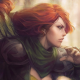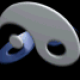Script by 3ICE.

• 03Dec

## All about colors (in Warcraft 3)

We can change the color of: Tooltips, Messages, Dialogs, Map names, Ability descriptions, and basically anything else that is identified as text (type: string) in Warcraft 3.

#### Hex numbers:

The hexadecimal system uses 16 numeric characters: `0 1 2 3 4 5 6 7 8 9 A B C D E F`
Numbers 0 through 9 are just like our regular numbers. Then there are 6 extra characters, represented by letters A through F. `A=10 B=11 C=12 D=13 E=14 F=15`

Using just two digits, you can express 256 different numbers in hex:

` 0=00 1=01 2=02 3=03 4=04 5=05 6=06 7=07 8=08 9=09 10=0A 11=0B 12=0C 13=0D 14=0E 15=0F 16=10 17=11 18=12 19=13 20=14 21=15 22=16 23=17 24=18 25=19 26=1A 27=1B 28=1C 29=1D 30=1E 31=1F 32=20 33=21 34=22 35=23 36=24 37=25 38=26 39=27 40=28 41=29 42=2A 43=2B 44=2C 45=2D 46=2E 47=2F 48=30 49=31 50=32 51=33 52=34 53=35 54=36 55=37 56=38 57=39 58=3A 59=3B 60=3C 61=3D 62=3E 63=3F 64=40 65=41 66=42 67=43 68=44 69=45 70=46 71=47 72=48 73=49 74=4A 75=4B 76=4C 77=4D 78=4E 79=4F 80=50 81=51 82=52 83=53 84=54 85=55 86=56 87=57 88=58 89=59 90=5A 91=5B 92=5C 93=5D 94=5E 95=5F 96=60 97=61 98=62 99=63 100=64 101=65 102=66 103=67 104=68 105=69 106=6A 107=6B 108=6C 109=6D 110=6E 111=6F112=70 113=71 114=72 115=73 116=74 117=75 118=76 119=77 120=78 121=79 122=7A 123=7B 124=7C 125=7D126=7E 127=7F 128=80 129=81 130=82 131=83 132=84 133=85 134=86 135=87 136=88 137=89 138=8A 139=8B140=8C 141=8D 142=8E 143=8F 144=90 145=91 146=92 147=93 148=94 149=95 150=96 151=97 152=98 153=99154=9A 155=9B 156=9C 157=9D 158=9E 159=9F 160=A0 161=A1 162=A2 163=A3 164=A4 165=A5 166=A6 167=A7168=A8 169=A9 170=AA 171=AB 172=AC 173=AD 174=AE 175=AF 176=B0 177=B1 178=B2 179=B3 180=B4 181=B5182=B6 183=B7 184=B8 185=B9 186=BA 187=BB 188=BC 189=BD 190=BE 191=BF 192=C0 193=C1 194=C2 195=C3196=C4 197=C5 198=C6 199=C7 200=C8 201=C9 202=CA 203=CB 204=CC 205=CD 206=CE 207=CF 208=D0 209=D1210=D2 211=D3 212=D4 213=D5 214=D6 215=D7 216=D8 217=D9 218=DA 219=DB 220=DC 221=DD 222=DE 223=DF224=E0 225=E1 226=E2 227=E3 228=E4 229=E5 230=E6 231=E7 232=E8 233=E9 234=EA 235=EB 236=EC 237=ED238=EE 239=EF 240=F0 241=F1 242=F2 243=F3 244=F4 245=F5 246=F6 247=F7 248=F8 249=F9 250=FA 251=FB252=FC 253=FD 254=FE 255=FF`

#### Building a color code:

A color code has the following parts:

• (4) The starting tag is always `|cff`. `|c` means start the color and `ff` would be the alpha value, but since texts cannot have transparency, these 2 characters are actually obsolete.
• (2) Red tone, determines how much red is in the color `00-ff`
• (2) Green tone, determines how much green is in the color `00-ff`
• (2) Blue tone, determines how much blue is in the color `00-ff`
• (x) The text itself, can be of any length.
• (2) The closing tag is `|r` it turns the text color back to white, can be omitted, acts exactly like `|cffffffff`.

In one sentence: Colors are applied with |cTTRRGGBBx|r, where |c starts the color, TT is useless, RR is red, GG is green, BB is blue, x is the colored text itself and |r stops the color.

#### Common Colors:

|cffff0000Red|r
|cff0000ffBlue|r
|cff00ffffTeal|r
|cff550088Purple|r
|cffffff00Yellow|r
|cffff8800Orange|r
|cff00ff00Green|r
|cffee55bbPink|r
|cff999999Gray|r
|cff77bbffLight Blue|r
|cff116644Dark Green|r
|cff552200Brown|r
and
|cffffcc00Hotkey (Gold color)|r

#### Rainbow colors:

Many people use programs for generating colorful texts because they can't or don't want to understand how warcraft works. You can get coloring tools from here.

#### Standards:

There is no official standard for color code format, I have seen them in lower-, upper- and even mixed-case. I suggest using lowercase hex codes, because all Blizzard-made tooltips are in lowercase and XHTML strictly enforces lowercase hex codes as well.

Posted by 3ICE @ 8:12 am

### 19 Responses•TheEnigmaBlade Says:

Awesome, i've been looking for the standard colors!!! Thank you so much!

•Zinnoc Says:

Thanks dude , i was looking very long for them , i need it for my maps , thank you so much. :]

•Infernal Says:

Thanks! I was searching for that for a long time. Now i can create my map.

•">ViCK Says:

Excellent tutorial. I thank you for listing the colors for Players 1-12.

What is "|cffffcc00Hotkey (Gold color)|r"? Is it Player 13? I though neutrals were gray-ish.

•">ViCK Says:

Never mind, I figured it out. It's for highlighting the hotkey in tooltips with a different color. I never did that before, but now that you taught me coloring, I will! Thanks again.

•Dead_Angel >. Says:

Tyvm! This is awesome. Always wondered how i can color my text.

•Thurst4blood Says:

Thanks 3ICE, I needed colour text in my new map im making it will be out in august.
Map Will Be Done on the 2/8/09.

Thurst4blood.

•The_Juggernaut Says:

This is sooooo useful :D I have used this information many times and I hope this page never disappears XD

•3ICE Says:

Dear The_Juggernaut,

I can promise you that this page will stay here for ever.

Or at least that this information will stay somewhere on my website for ever. As I'm working on the code for a new website, which will replace both my blog and forum, merging them into one system. I need to come up with a good name for it though, something like: forumblog forumlog forlog folog flog blogforum blogorum blogrum blorum blom blum - none of these are good enough.

–3ICE

•Thurst4blood Says:

Hi 3ICE,

I am planning on making a Maze/skate map, and I have nearly all the trigger i require :), but there is one I still don't know..

Could you help me? The trigger I need is the Name Changer trigger. =)

Please if you know the trigger :)

•3ICE Says:

I hope you realize that you posted a trigger question on a blog post about color codes. I have a forum, remember? Go there, ask there. Link is in the top-left sidebar under #3.

•Thurst4blood Says:

OK :) thanks.

•zXcongducXz Says:

Try my code wrote by HTML languages
http://www.mediafire.com/?2cmfaytar48rx

if s^m1 wanna create new map then i may help
pm this email:
zXcongducXz@yahoo.com.vn

•3ICE Says:

Thanks for your contribution, it works very well.

Could you change all your uppercase color codes to lowercase? That is the "standard".
(|cffFFCC00 –> |cffffcc00)

–3ICE

•zXcongducXz Says:

http://www.mediafire.com/?2cmfaytar48rx (if that file not exists)

•Moneybag Says:

Just thought you should know, I have this site bookmarked :) wuv you and thanks!

•Aiciel Says:

3ICE, Forgive me if I sound stupid since everyone else seems to understand how to implement this, but I am kind of new to this. I understand what you explained above, however I am not sure where I am supposed to type that in my world editor for Warcraft III. I have a guess but I am not completely sure that it is right. If I wanted make an item called Chain Mail then all I would have to do is go to rename under the object editor and type in |cffffcc00Chain Mail|r in place of its name?

•3ICE Says:

Yes, if you want to color the item's name gold, then that would be the perfect solution. It produces: `Chain Mail`
(I usually color my item names gray/white/green/blue/purple/orange based on their quality, like World of Warcraft) Gold is usually reserved for showing the hotkey letter.

For example if you want the hotkey for purchasing the item to be C and signify that with a gold highlight (as is the Blizzard standard), the item's name would remain `Chain Mail` but it's tooltip would be: `Purchase |cffffcc00C|rhain Mail`
This produces: `Purchase Chain Mail`

Note that you don't need to color item names. Plain white is good enough in most cases, but special items usually get colorful names. Item names themselves don't usually carry color information. Only the tooltip.

•Aiciel Says:

Thanks so much, that's very helpful to know.